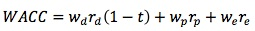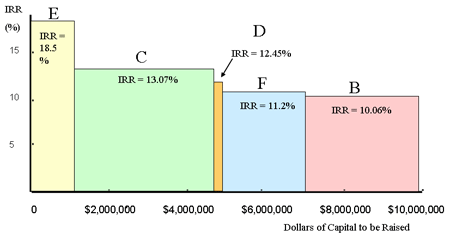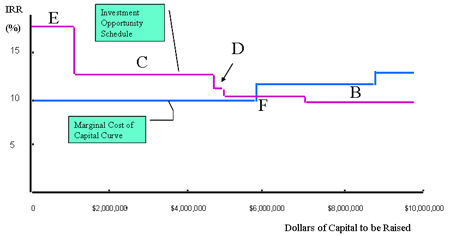### Why should I choose AnalystNotes?

AnalystNotes specializes in helping candidates pass. Period.

##### Subject 1. Cost of Capital
Capital is a necessary factor of production, and has a cost. The providers of capital require a return on their money. A firm must ensure that stockholders or those that have lent the firm money (such as banks) receive the return that they require. This return is the cost that the firm will incur to maintain those sources of capital. Therefore, the return that the providers of funds require is equal to the cost to the firm of maintaining those funds.

Calculating the cost of capital is important for a firm, as this is the rate of return that must be used when evaluating capital projects. The return from the project must be greater than the cost of the project in order for it to be acceptable.

In general, a firm can finance its operations from three main sources of capital:

• equity or common stock
• preferred stock
• debt

Each of these sources of capital has a cost. The cost of capital used in capital budgeting should be calculated as a weighted average, or composite, of the various types of funds a firm generally uses.• The weighted average cost of capital (WACC) is defined as the weighted average cost of the component costs of debt, preferred stock, and common stock or equity. It is also referred to as the marginal cost of capital (MCC), which is the cost of obtaining another dollar of new capital.
• wd = the weight for debt
• wp = the weight for preferred stock
• we = the weight for common stock
• r = required rate for each component
• t = the marginal tax rate

Taxes and the Cost of Capital

Interest on debt is tax deductible; therefore, to calculate the cost of debt, the tax benefit is deducted. This means that after-tax cost of debt = interest rate - tax savings (the government pays part of the cost of debt as interest is tax deductible).

There is no tax savings associated with the use of preferred stock or common stock.

Weights of the Weighted Average

The target capital structure is the percentage of debt, preferred stock, and common equity that a firm is striving to maintain and that will maximize the firm's stock price. Each firm has a target capital structure, and it should raise new capital in a manner that will keep the actual capital structure on target over time.

• If the target capital structure is known, it should be used.
• If not, market values of debt and stocks should be used to calculate weights. That is, the company's current capital structure is assumed to represent the company's target capital structure.
• If this is not possible, then trends in the company's capital structure or averages of comparable companies' capital structures should be used as targets.

Example

Firm A has a capital structure consisting of 40% debt, 5% preferred stock, and 55% common equity (made up of retained earnings and common stock). Firm A pays 10% interest on its debt and has a marginal tax rate of 35%. If Firm A's component cost of preferred stock is 12.5% and the component cost of common stock equity from retained earnings is 13.5%, calculate Firm A's WACC.

WACC = 0.4 x 10% (1 - 0.35) + 0.05 x 12.5% + 0.55 x 13.5% = 0.026 + 0.00625 + 0.07425 = 10.65%

Investment Opportunity Schedule

In any one year, a firm may consider a number of capital projects. The greater the number of projects undertaken, the more money the firm will have to raise in order to finance them.

There is a limit to the amount of money that can be raised in any one year (i.e., the capital markets are finite, and there is a limit to the number of investors and the amount of funds available to invest in any prospect in any given year.) Hence it is important that the capital budgeting analysis be extended to take this fact into account.

The Investment Opportunity Schedule (IOS) is the prioritized list of capital projects, listed by IRR (internal rate of return) from highest to lowest. The cumulative investment required is also listed.

Example

Consider a firm that has six different capital investment proposals this year. Each project has its own IRR and capital cost. Each project has the same risk as the firm as a whole.The first step in developing an IOS is to order the projects from highest to lowest IRR, and then calculate the cumulative capital cost of the projects.First, it is clear that project A is unacceptable: it offers a rate of return (IRR) that is less than the firm's cost of capital.

The remaining projects certainly meet the first investment screen (they have positive NPVs; they offer rates of return in excess of the firm's WACC).

Now a graphical representation of the IOS can be prepared by plotting the projects' IRRs against the cumulative dollars of capital to be raised.The height of each cylinder is equal to the project's IRR; the width is equal to the initial investment for the project.As a firm raises more and more capital in any one year, it will exhaust the most readily available (and lowest cost) sources first. If the firm has to raise further capital, it will cost the firm more and the MCC will rise. In this case, project B would definitely be rejected. F is very marginal.The optimal capital budget is that amount of capital raised and invested at which the MCC = marginal return from investing.

Learning Outcome Statements

a. calculate and interpret the weighted average cost of capital (WACC) of a company;

b. describe how taxes affect the cost of capital from different capital sources;

c. describe the use of target capital structure in estimating WACC and how target capital structure weights may be determined;

d. explain how the marginal cost of capital and the investment opportunity schedule are used to determine the optimal capital budget;

e. explain the marginal cost of capital's role in determining the net present value of a project;

CFA® 2021 Level I Curriculum, , Volume 4, Reading 33

User Comment
DariSH why not ranking projects by NPV?
shiva5555 Because some cost more, so you can make more in quantity but you want the highest returns to make the most on limited funds.
johntan1979 How to calculate the cumulative cost of capital of the projects? Do we need to know how?
johntan1979 Oh! Nevermind... just realized I asked a stupid question... Cumulative cost of capital means the cumulative initial costs... Duh! (to myself)
kononen2 Where is this WACCe and WACCs from the quizz Q14? Found MCC in the last sentence here... Can't find WACCe/WACCs
willhc03 This example is a bit problematic in my opinion. The problem is that NPV and IRR conflict for several of these projects. This means that NPV should be considered. The solution above is technically correct, but very specific to this example. If MCC jumps at \$6m invested as in this example, then Projects E, C, and D are value maximizing. However, if the firm can invest \$7m at 10%, the method above would have you choose the same three projects for a total NPV of 581,199. However, the correct budgeting decision is to accept C, E, and F for a total NPV of 661,133, an additional 79,934 in value created. For an example like this, here is a suggestion:
1. Rank projects by NPV
2. Accept projects in decreasing NPV order until the point where marginal cost of capital begins to increase
3. Look for any additional projects with lower NPV, but can be undertaken without raising marginal cost of capital. Choose among those in decreasing NPV order.
The example is illustrating the optimal capital budget where the blue line (WACC / MCC) intersect the purple line at F.

We must consider to undertake projects that has IRRs greater than the optimal capital budget.

Why do we have to compare WACC (MCC) with IRR but not with NPV?
because if IRR < WACC => negative NPV.